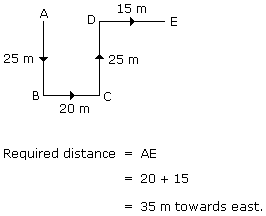# Verbal Reasoning - Direction Sense Test - Discussion

### Discussion :: Direction Sense Test - Direction Sense 1 (Q.No.32)

32.

Rohit walked 25 m towards south. Then he turned to his left and walked 20 m. He then turned to his left and walked 25 m. He again turned to his right and walked 15 m. At what distance is he from the starting point and in which direction?

 [A]. 35 m East [B]. 35 m North [C]. 30 m West [D]. 45 m East

Explanation:123 said: (Nov 28, 2013) How 35 is calculated?

 Gong Doni said: (Jun 13, 2017) Yeah, really want to know how 35 is calculated?

 Pallavi Singh said: (Jul 14, 2017) According to figure. BC & AD is the same distance 20 m and then added the distance of DE so the distance of AE is 35 m.

 Anupam said: (Aug 20, 2017) Total distance has to cover A to e in a straight line. So DE= 15 AD= distance missing. But AD distance = Bc distance= 10. so we can find the total distance from A to e. 15+ 20= 35.